# 1.5 Substitution  (Page 4/6)

 Page 4 / 6

$\int x\sqrt{4{x}^{2}+9}dx=\frac{1}{12}{\left(4{x}^{2}+9\right)}^{3\text{/}2}+C;u=4{x}^{2}+9$

$\int \frac{x}{\sqrt{4{x}^{2}+9}}dx=\frac{1}{4}\sqrt{4{x}^{2}+9}+C;u=4{x}^{2}+9$

$du=8xdx;f\left(u\right)=\frac{1}{8\sqrt{u}}$

$\int \frac{x}{{\left(4{x}^{2}+9\right)}^{2}}dx=-\frac{1}{8\left(4{x}^{2}+9\right)};u=4{x}^{2}+9$

In the following exercises, find the antiderivative using the indicated substitution.

$\int {\left(x+1\right)}^{4}dx;u=x+1$

$\frac{1}{5}{\left(x+1\right)}^{5}+C$

$\int {\left(x-1\right)}^{5}dx;u=x-1$

$\int {\left(2x-3\right)}^{-7}dx;u=2x-3$

$-\frac{1}{12{\left(3-2x\right)}^{6}}+C$

$\int {\left(3x-2\right)}^{-11}dx;u=3x-2$

$\int \frac{x}{\sqrt{{x}^{2}+1}}dx;u={x}^{2}+1$

$\sqrt{{x}^{2}+1}+C$

$\int \frac{x}{\sqrt{1-{x}^{2}}}dx;u=1-{x}^{2}$

$\int \left(x-1\right){\left({x}^{2}-2x\right)}^{3}dx;u={x}^{2}-2x$

$\frac{1}{8}{\left({x}^{2}-2x\right)}^{4}+C$

$\int \left({x}^{2}-2x\right){\left({x}^{3}-3{x}^{2}\right)}^{2}dx;u={x}^{3}=3{x}^{2}$

$\int {\text{cos}}^{3}\theta d\theta ;u=\text{sin}\phantom{\rule{0.1em}{0ex}}\theta$ $\text{(}Hint\text{:}\phantom{\rule{0.2em}{0ex}}{\text{cos}}^{2}\theta =1-{\text{sin}}^{2}\theta \text{)}$

$\text{sin}\phantom{\rule{0.1em}{0ex}}\theta -\frac{{\text{sin}}^{3}\theta }{3}+C$

$\int {\text{sin}}^{3}\theta d\theta ;u=\text{cos}\phantom{\rule{0.1em}{0ex}}\theta$ $\text{(}Hint\text{:}\phantom{\rule{0.2em}{0ex}}{\text{sin}}^{2}\theta =1-{\text{cos}}^{2}\theta \text{)}$

In the following exercises, use a suitable change of variables to determine the indefinite integral.

$\int x{\left(1-x\right)}^{99}dx$

$\frac{{\left(1-x\right)}^{101}}{101}-\frac{{\left(1-x\right)}^{100}}{100}+C$

$\int t{\left(1-{t}^{2}\right)}^{10}dt$

$\int {\left(11x-7\right)}^{-3}dx$

$-\frac{1}{22\left(7-11{x}^{2}\right)}+C$

$\int {\left(7x-11\right)}^{4}dx$

$\int {\text{cos}}^{3}\theta \phantom{\rule{0.1em}{0ex}}\text{sin}\phantom{\rule{0.1em}{0ex}}\theta d\theta$

$-\frac{{\text{cos}}^{4}\theta }{4}+C$

$\int {\text{sin}}^{7}\theta \phantom{\rule{0.1em}{0ex}}\text{cos}\phantom{\rule{0.1em}{0ex}}\theta d\theta$

$\int {\text{cos}}^{2}\left(\pi t\right)\text{sin}\left(\pi t\right)dt$

$-\frac{{\text{cos}}^{3}\left(\pi t\right)}{3\pi }+C$

$\int {\text{sin}}^{2}x{\text{cos}}^{3}xdx$ $\text{(}Hint\text{:}\phantom{\rule{0.2em}{0ex}}{\text{sin}}^{2}x+{\text{cos}}^{2}x=1\text{)}$

$\int t\phantom{\rule{0.1em}{0ex}}\text{sin}\left({t}^{2}\right)\text{cos}\left({t}^{2}\right)dt$

$-\frac{1}{4}\phantom{\rule{0.05em}{0ex}}{\text{cos}}^{2}\left({t}^{2}\right)+C$

$\int {t}^{2}{\text{cos}}^{2}\left({t}^{3}\right)\text{sin}\left({t}^{3}\right)dt$

$\int \frac{{x}^{2}}{{\left({x}^{3}-3\right)}^{2}}dx$

$-\frac{1}{3\left({x}^{3}-3\right)}+C$

$\int \frac{{x}^{3}}{\sqrt{1-{x}^{2}}}dx$

$\int \frac{{y}^{5}}{{\left(1-{y}^{3}\right)}^{3\text{/}2}}dy$

$-\frac{2\left({y}^{3}-2\right)}{3\sqrt{1-{y}^{3}}}$

${\int \text{cos}\phantom{\rule{0.1em}{0ex}}\theta \left(1-\text{cos}\phantom{\rule{0.1em}{0ex}}\theta \right)}^{99}\text{sin}\phantom{\rule{0.1em}{0ex}}\theta d\theta$

${\int \left(1-{\text{cos}}^{3}\theta \right)}^{10}{\text{cos}}^{2}\theta \phantom{\rule{0.1em}{0ex}}\text{sin}\phantom{\rule{0.1em}{0ex}}\theta d\theta$

$\frac{1}{33}{\left(1-{\text{cos}}^{3}\theta \right)}^{11}+C$

$\int \left(\text{cos}\phantom{\rule{0.1em}{0ex}}\theta -1\right){\left({\text{cos}}^{2}\theta -2\phantom{\rule{0.1em}{0ex}}\text{cos}\phantom{\rule{0.1em}{0ex}}\theta \right)}^{3}\text{sin}\phantom{\rule{0.1em}{0ex}}\theta d\theta$

$\int \left({\text{sin}}^{2}\theta -2\phantom{\rule{0.1em}{0ex}}\text{sin}\phantom{\rule{0.1em}{0ex}}\theta \right){\left({\text{sin}}^{3}\theta -3{\text{sin}}^{2}\theta \right)}^{3}\text{cos}\phantom{\rule{0.1em}{0ex}}\theta d\theta$

$\frac{1}{12}{\left({\text{sin}}^{3}\theta -3{\text{sin}}^{2}\theta \right)}^{4}+C$

In the following exercises, use a calculator to estimate the area under the curve using left Riemann sums with 50 terms, then use substitution to solve for the exact answer.

[T] $y=3{\left(1-x\right)}^{2}$ over $\left[0,2\right]$

[T] $y=x{\left(1-{x}^{2}\right)}^{3}$ over $\left[-1,2\right]$

${L}_{50}=-8.5779.$ The exact area is $\frac{-81}{8}$

[T] $y=\text{sin}\phantom{\rule{0.1em}{0ex}}x{\left(1-\text{cos}\phantom{\rule{0.1em}{0ex}}x\right)}^{2}$ over $\left[0,\pi \right]$

[T] $y=\frac{x}{{\left({x}^{2}+1\right)}^{2}}$ over $\left[-1,1\right]$

${L}_{50}=-0.006399$ … The exact area is 0.

In the following exercises, use a change of variables to evaluate the definite integral.

${\int }_{0}^{1}x\sqrt{1-{x}^{2}}dx$

${\int }_{0}^{1}\frac{x}{\sqrt{1+{x}^{2}}}dx$

$u=1+{x}^{2},du=2xdx,\frac{1}{2}{\int }_{1}^{2}{u}^{-1\text{/}2}du=\sqrt{2}-1$

${\int }_{0}^{2}\frac{t}{\sqrt{5+{t}^{2}}}dt$

${\int }_{0}^{1}\frac{t}{\sqrt{1+{t}^{3}}}dt$

$u=1+{t}^{3},du=3{t}^{2},\frac{1}{3}{\int }_{1}^{2}{u}^{-1\text{/}2}du=\frac{2}{3}\left(\sqrt{2}-1\right)$

${\int }_{0}^{\pi \text{/}4}{\text{sec}}^{2}\theta \phantom{\rule{0.1em}{0ex}}\text{tan}\phantom{\rule{0.1em}{0ex}}\theta d\theta$

${\int }_{0}^{\pi \text{/}4}\frac{\text{sin}\phantom{\rule{0.1em}{0ex}}\theta }{{\text{cos}}^{4}\theta }d\theta$

$u=\text{cos}\phantom{\rule{0.1em}{0ex}}\theta ,du=\text{−}\text{sin}\phantom{\rule{0.1em}{0ex}}\theta d\theta ,{\int }_{1\text{/}\sqrt{2}}^{1}{u}^{-4}du=\frac{1}{3}\left(2\sqrt{2}-1\right)$

In the following exercises, evaluate the indefinite integral $\int f\left(x\right)dx$ with constant $C=0$ using u -substitution. Then, graph the function and the antiderivative over the indicated interval. If possible, estimate a value of C that would need to be added to the antiderivative to make it equal to the definite integral $F\left(x\right)={\int }_{a}^{x}f\left(t\right)dt,$ with a the left endpoint of the given interval.

[T] $\int \left(2x+1\right){e}^{{x}^{2}+x-6}dx$ over $\left[-3,2\right]$

[T] $\int \frac{\text{cos}\left(\text{ln}\left(2x\right)\right)}{x}dx$ on $\left[0,2\right]$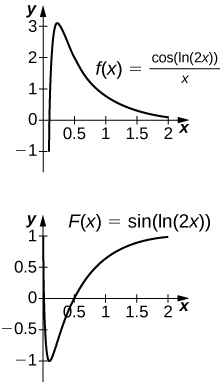The antiderivative is $y=\text{sin}\left(\text{ln}\left(2x\right)\right).$ Since the antiderivative is not continuous at $x=0,$ one cannot find a value of C that would make $y=\text{sin}\left(\text{ln}\left(2x\right)\right)-C$ work as a definite integral.

[T] $\int \frac{3{x}^{2}+2x+1}{\sqrt{{x}^{3}+{x}^{2}+x+4}}dx$ over $\left[-1,2\right]$

[T] $\int \frac{\text{sin}\phantom{\rule{0.1em}{0ex}}x}{{\text{cos}}^{3}x}dx$ over $\left[-\frac{\pi }{3},\frac{\pi }{3}\right]$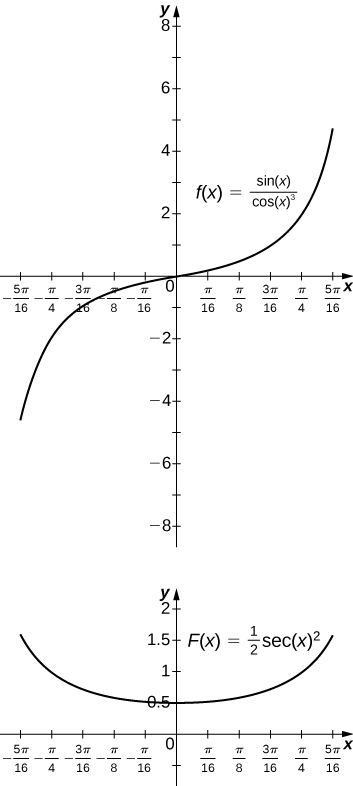The antiderivative is $y=\frac{1}{2}\phantom{\rule{0.05em}{0ex}}{\text{sec}}^{2}x.$ You should take $C=-2$ so that $F\left(-\frac{\pi }{3}\right)=0.$

[T] $\int \left(x+2\right){e}^{\text{−}{x}^{2}-4x+3}dx$ over $\left[-5,1\right]$

[T] $\int 3{x}^{2}\sqrt{2{x}^{3}+1}dx$ over $\left[0,1\right]$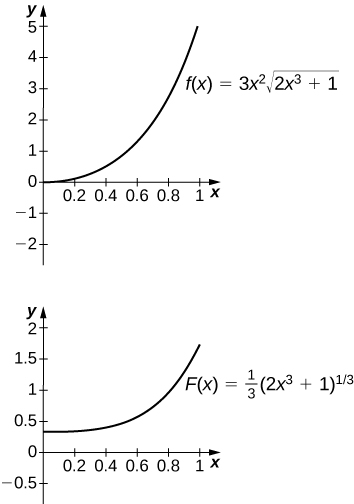The antiderivative is $y=\frac{1}{3}{\left(2{x}^{3}+1\right)}^{3\text{/}2}.$ One should take $C=-\frac{1}{3}.$

If $h\left(a\right)=h\left(b\right)$ in ${\int }_{a}^{b}g\text{'}\left(h\left(x\right)\right)h\left(x\right)dx,$ what can you say about the value of the integral?

Is the substitution $u=1-{x}^{2}$ in the definite integral ${\int }_{0}^{2}\frac{x}{1-{x}^{2}}dx$ okay? If not, why not?

No, because the integrand is discontinuous at $x=1.$

In the following exercises, use a change of variables to show that each definite integral is equal to zero.

${\int }_{0}^{\pi }{\text{cos}}^{2}\left(2\theta \right)\text{sin}\left(2\theta \right)d\theta$

${\int }_{0}^{\sqrt{\pi }}t\phantom{\rule{0.1em}{0ex}}\text{cos}\left({t}^{2}\right)\text{sin}\left({t}^{2}\right)dt$

$u=\text{sin}\left({t}^{2}\right);$ the integral becomes $\frac{1}{2}{\int }_{0}^{0}udu.$

${\int }_{0}^{1}\left(1-2t\right)dt$

${\int }_{0}^{1}\frac{1-2t}{\left(1+{\left(t-\frac{1}{2}\right)}^{2}\right)}dt$

$u=\left(1+{\left(t-\frac{1}{2}\right)}^{2}\right);$ the integral becomes $\text{−}{\int }_{5\text{/}4}^{5\text{/}4}\frac{1}{u}du.$

${\int }_{0}^{\pi }\text{sin}\left({\left(t-\frac{\pi }{2}\right)}^{3}\right)\text{cos}\left(t-\frac{\pi }{2}\right)dt$

${\int }_{0}^{2}\left(1-t\right)\text{cos}\left(\pi t\right)dt$

$u=1-t;$ the integral becomes
$\begin{array}{l}{\int }_{1}^{-1}u\phantom{\rule{0.1em}{0ex}}\text{cos}\left(\pi \left(1-u\right)\right)du\hfill \\ ={\int }_{1}^{-1}u\left[\text{cos}\phantom{\rule{0.1em}{0ex}}\pi \phantom{\rule{0.1em}{0ex}}\text{cos}\phantom{\rule{0.1em}{0ex}}u-\text{sin}\phantom{\rule{0.1em}{0ex}}\pi \phantom{\rule{0.1em}{0ex}}\text{sin}\phantom{\rule{0.1em}{0ex}}u\right]du\hfill \\ =\text{−}{\int }_{1}^{-1}u\phantom{\rule{0.1em}{0ex}}\text{cos}\phantom{\rule{0.1em}{0ex}}udu\hfill \\ ={\int }_{-1}^{1}u\phantom{\rule{0.1em}{0ex}}\text{cos}\phantom{\rule{0.1em}{0ex}}udu=0\hfill \end{array}$
since the integrand is odd.

${\int }_{\pi \text{/}4}^{3\pi \text{/}4}{\text{sin}}^{2}t\phantom{\rule{0.1em}{0ex}}\text{cos}\phantom{\rule{0.1em}{0ex}}tdt$

Show that the average value of $f\left(x\right)$ over an interval $\left[a,b\right]$ is the same as the average value of $f\left(cx\right)$ over the interval $\left[\frac{a}{c},\frac{b}{c}\right]$ for $c>0.$

Setting $u=cx$ and $du=cdx$ gets you $\frac{1}{\frac{b}{c}-\frac{a}{c}}{\int }_{a\text{/}c}^{b\text{/}c}f\left(cx\right)dx=\frac{c}{b-a}\phantom{\rule{0.2em}{0ex}}{\int }_{u=a}^{u=b}f\left(u\right)\frac{du}{c}=\frac{1}{b-a}{\int }_{a}^{b}f\left(u\right)du.$

Find the area under the graph of $f\left(t\right)=\frac{t}{{\left(1+{t}^{2}\right)}^{a}}$ between $t=0$ and $t=x$ where $a>0$ and $a\ne 1$ is fixed, and evaluate the limit as $x\to \infty .$

Find the area under the graph of $g\left(t\right)=\frac{t}{{\left(1-{t}^{2}\right)}^{a}}$ between $t=0$ and $t=x,$ where $0 and $a>0$ is fixed. Evaluate the limit as $x\to 1.$

${\int }_{0}^{x}g\left(t\right)dt=\frac{1}{2}{\int }_{u=1-{x}^{2}}^{1}\frac{du}{{u}^{a}}=\frac{1}{2\left(1-a\right)}{u}^{1-a}{|}_{u=1-{x}^{2}}^{1}=\frac{1}{2\left(1-a\right)}\left(1-{\left(1-{x}^{2}\right)}^{1-a}\right).$ As $x\to 1$ the limit is $\frac{1}{2\left(1-a\right)}$ if $a<1,$ and the limit diverges to +∞ if $a>1.$

The area of a semicircle of radius 1 can be expressed as ${\int }_{-1}^{1}\sqrt{1-{x}^{2}}dx.$ Use the substitution $x=\text{cos}\phantom{\rule{0.1em}{0ex}}t$ to express the area of a semicircle as the integral of a trigonometric function. You do not need to compute the integral.

The area of the top half of an ellipse with a major axis that is the x -axis from $x=-1$ to a and with a minor axis that is the y -axis from $y=\text{−}b$ to b can be written as ${\int }_{\text{−}a}^{a}b\sqrt{1-\frac{{x}^{2}}{{a}^{2}}}dx.$ Use the substitution $x=a\phantom{\rule{0.1em}{0ex}}\text{cos}\phantom{\rule{0.1em}{0ex}}t$ to express this area in terms of an integral of a trigonometric function. You do not need to compute the integral.

${\int }_{t=\pi }^{0}b\sqrt{1-{\text{cos}}^{2}t}\phantom{\rule{0.2em}{0ex}}×\phantom{\rule{0.2em}{0ex}}\left(\text{−}a\phantom{\rule{0.1em}{0ex}}\text{sin}\phantom{\rule{0.1em}{0ex}}t\right)dt={\int }_{t=0}^{\pi }ab{\text{sin}}^{2}tdt$

[T] The following graph is of a function of the form $f\left(t\right)=a\phantom{\rule{0.1em}{0ex}}\text{sin}\left(nt\right)+b\phantom{\rule{0.1em}{0ex}}\text{sin}\left(mt\right).$ Estimate the coefficients a and b , and the frequency parameters n and m . Use these estimates to approximate ${\int }_{0}^{\pi }f\left(t\right)dt.$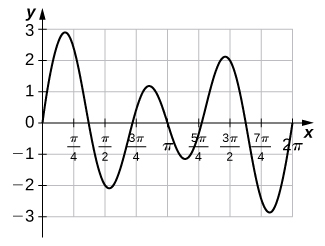[T] The following graph is of a function of the form $f\left(x\right)=a\phantom{\rule{0.1em}{0ex}}\text{cos}\left(nt\right)+b\phantom{\rule{0.1em}{0ex}}\text{cos}\left(mt\right).$ Estimate the coefficients a and b and the frequency parameters n and m . Use these estimates to approximate ${\int }_{0}^{\pi }f\left(t\right)dt.$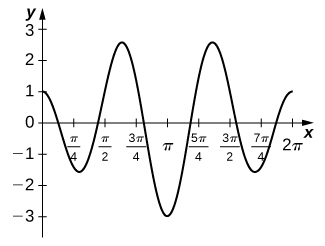$f\left(t\right)=2\phantom{\rule{0.1em}{0ex}}\text{cos}\left(3t\right)-\text{cos}\left(2t\right);{\int }_{0}^{\pi \text{/}2}\left(2\phantom{\rule{0.1em}{0ex}}\text{cos}\left(3t\right)-\text{cos}\left(2t\right)\right)=-\frac{2}{3}$

where we get a research paper on Nano chemistry....?
what are the products of Nano chemistry?
There are lots of products of nano chemistry... Like nano coatings.....carbon fiber.. And lots of others..
learn
Even nanotechnology is pretty much all about chemistry... Its the chemistry on quantum or atomic level
learn
da
no nanotechnology is also a part of physics and maths it requires angle formulas and some pressure regarding concepts
Bhagvanji
Preparation and Applications of Nanomaterial for Drug Delivery
revolt
da
Application of nanotechnology in medicine
what is variations in raman spectra for nanomaterials
I only see partial conversation and what's the question here!
what about nanotechnology for water purification
please someone correct me if I'm wrong but I think one can use nanoparticles, specially silver nanoparticles for water treatment.
Damian
yes that's correct
Professor
I think
Professor
Nasa has use it in the 60's, copper as water purification in the moon travel.
Alexandre
nanocopper obvius
Alexandre
what is the stm
is there industrial application of fullrenes. What is the method to prepare fullrene on large scale.?
Rafiq
industrial application...? mmm I think on the medical side as drug carrier, but you should go deeper on your research, I may be wrong
Damian
How we are making nano material?
what is a peer
What is meant by 'nano scale'?
What is STMs full form?
LITNING
scanning tunneling microscope
Sahil
how nano science is used for hydrophobicity
Santosh
Do u think that Graphene and Fullrene fiber can be used to make Air Plane body structure the lightest and strongest. Rafiq
Rafiq
what is differents between GO and RGO?
Mahi
what is simplest way to understand the applications of nano robots used to detect the cancer affected cell of human body.? How this robot is carried to required site of body cell.? what will be the carrier material and how can be detected that correct delivery of drug is done Rafiq
Rafiq
if virus is killing to make ARTIFICIAL DNA OF GRAPHENE FOR KILLED THE VIRUS .THIS IS OUR ASSUMPTION
Anam
analytical skills graphene is prepared to kill any type viruses .
Anam
Any one who tell me about Preparation and application of Nanomaterial for drug Delivery
Hafiz
what is Nano technology ?
write examples of Nano molecule?
Bob
The nanotechnology is as new science, to scale nanometric
brayan
nanotechnology is the study, desing, synthesis, manipulation and application of materials and functional systems through control of matter at nanoscale
Damian
Is there any normative that regulates the use of silver nanoparticles?
what king of growth are you checking .?
Renato
What fields keep nano created devices from performing or assimulating ? Magnetic fields ? Are do they assimilate ?
why we need to study biomolecules, molecular biology in nanotechnology?
?
Kyle
yes I'm doing my masters in nanotechnology, we are being studying all these domains as well..
why?
what school?
Kyle
biomolecules are e building blocks of every organics and inorganic materials.
Joe
how did you get the value of 2000N.What calculations are needed to arrive at it
Privacy Information Security Software Version 1.1a
Good
Leaves accumulate on the forest floor at a rate of 2 g/cm2/yr and also decompose at a rate of 90% per year. Write a differential equation governing the number of grams of leaf litter per square centimeter of forest floor, assuming at time 0 there is no leaf litter on the ground. Does this amount approach a steady value? What is that value?
You have a cup of coffee at temperature 70°C, which you let cool 10 minutes before you pour in the same amount of milk at 1°C as in the preceding problem. How does the temperature compare to the previous cup after 10 minutes?
AbdulBy OpenStaxBy Richley CrapoBy Richley CrapoBy Richley CrapoBy Ryan LoweBy OpenStaxBy Sam LuongBy Michael SagByBy Dravida Mahadeo-J...